# RD Sharma Solutions Chapter 14 Coordinate Geometry Exercise 14.2 Class 10 Maths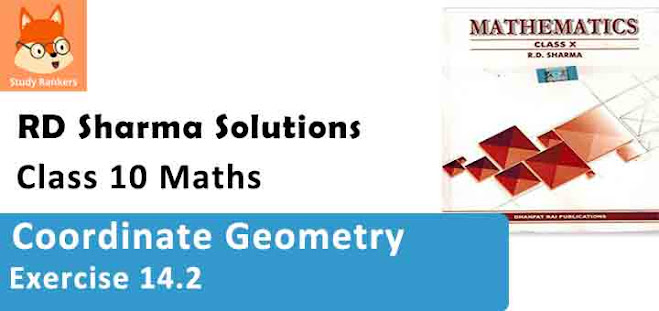Chapter Name RD Sharma Chapter 14 Coordinate Geometry Book Name RD Sharma Mathematics for Class 10 Other Exercises Exercise 14.1Exercise 14.2Exercise 14.3Exercise 14.4Exercise 14.5VSAQs and MCQs Related Study NCERT Solutions for Class 10 Maths

### Exercise 14.2 Solutions

1. Find the distance between the following pair of points :

(i) (-6, 7) and (-1, -5)
(ii) (a + b, b + c) and (a – b, c – b)
(iii) (a sin α, -b cos α) and (-a cos α, -b sin α)
(iv) (a, 0) and (0, b)

Solution

We know that the distance between two points A(x1, y1) and B(x2, y2) is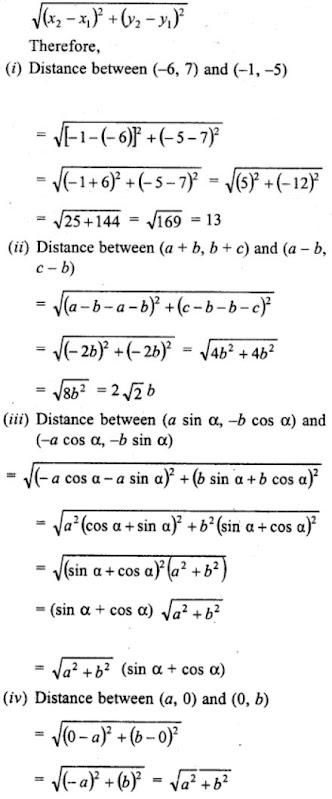2. Find the value of a when the distance between the points (3, a) and (4, 1) is √10

Solution

Distance between (3, a) and (4, 1).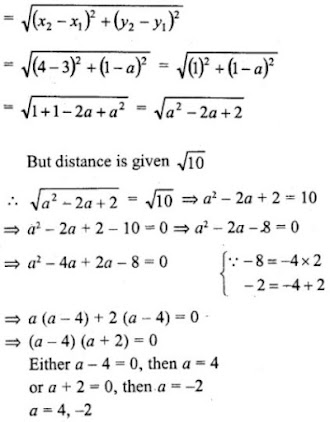3. If the points (2, 1) and (1, -2) are equidistant from the point (x, y), show that x + 3y = 0.

Solution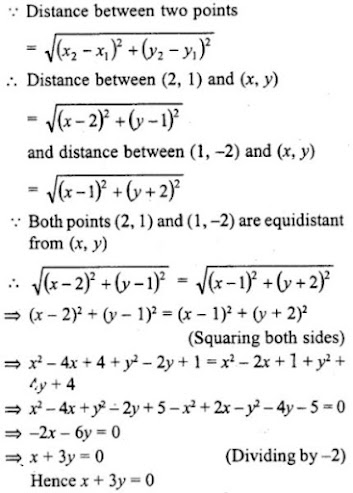4. Find the values of x, y if the distances of the point (x, y) from (-3, 0) as well as from (3, 0) are 4.

Solution

Distance between (x, y) and (-3, 0) is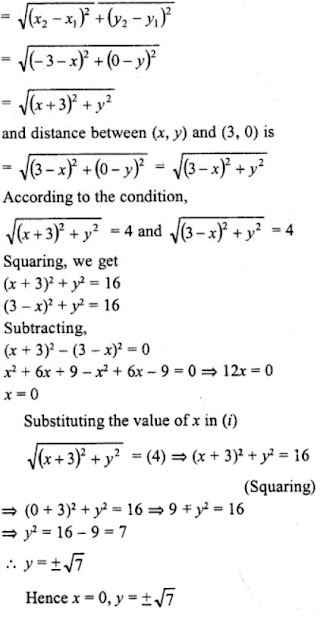5. The length of a line segment is of 10 units and the coordinates of one end-point are (2, -3). If the abscissa of the other end is 10, find the ordinate of the other end.

Solution

Let the ordinate of other end by y, then the distance between (2, -3) and (10, y) is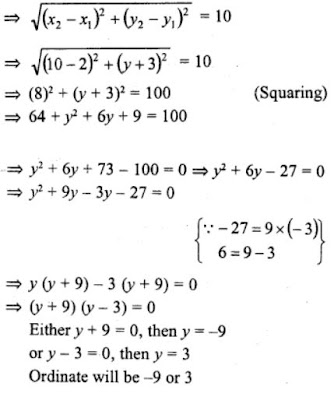6. Show that the points (-4, -1), (-2, -4), (4, 0) and (2, 3) are the vertices points of a rectangle.

Solution

Let ABCD is a rectangle whose vertices are:
A(-4, -1), B(-2, -4), C(4, 0) and D(2, 3)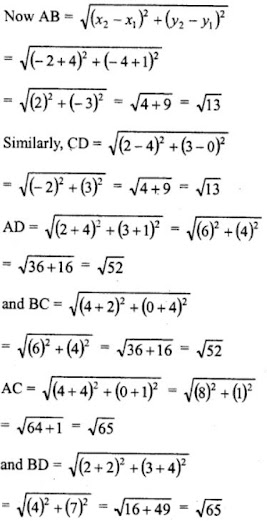AB = CD and AD = BC
and diagonal AC = BD
ABCD is a rectangle

7. Show that the points A (1, -2), B (3, 6), C (5, 10) and D (3, 2) are the vertices of a parallelogram.

Solution

Points are A(1, -2), B(3, 6), C(5, 10) and D(3,2).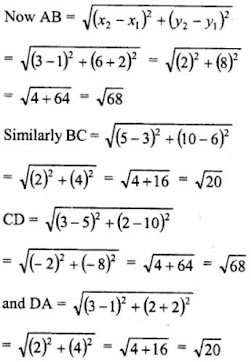∵ AB = CD and AD = BC
∴ ABCD is a parallelogram.

8. Prove that the points A (1, 7), B (4, 2), C (-1, -1) and D (-4, 4) are the vertices of a square.

Solution

Vertices A (1, 7), B (4, 2), C (-1,-1), D (-4, 4)
If these are the vertices of a square, then its diagonals and sides are equal.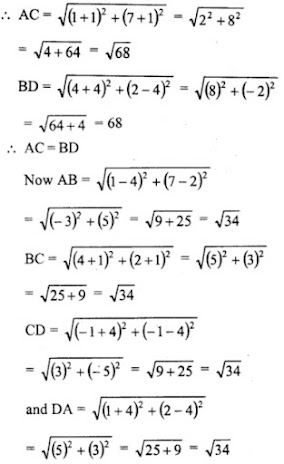∵ AB = BC = CD = DA and AC = BD
∴ ABCD is a square.

9. Prove that the points (3, 0), (6, 4) and (-1, 3) are the vertices of a right-angled isosceles triangle.

Solution

Vertices of ΔABC are A(3, 0), B(6, 4) and C(-1, 3)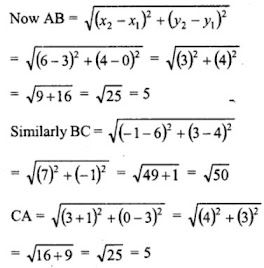We see that, AB = CA and BC is the longest side.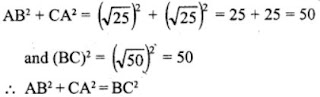Here, ΔABC is an isosceles right triangle.

10. Prove that (2, -2), (-2, 1) and (5, 2) are the vertices of a right angled triangle. Find the area of the triangle and the length of the hypotenuse.

Solution

Let the vertices of a triangle are A(2, -2), B(-2, 1) and C(5, 2)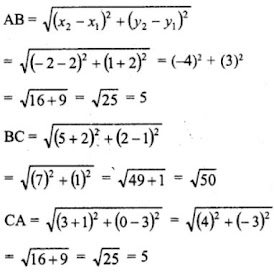We see that, AB = CA and BC is the longest side and AB2 + CA2 = 52 + 52 = 25 + 25 = 50 = BC2
ABC is a right angles triangle.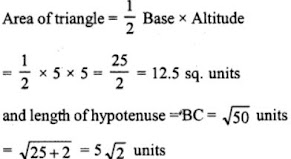11. Prove that the points (2a, 4a), (2a, 6a) and (2a + √3a , 5a) are the vertices of an equilateral triangle.

Solution

Vertices of ΔABC are A(2a, 4a), B(2a, 6a) and C(2a+ √3a, 5a)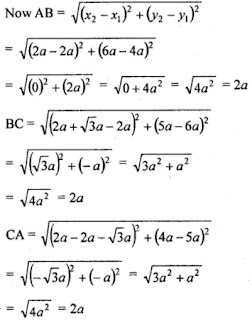∵ AB = BC = CA = 2a and an equilateral triangle has three equal sides.
∴ ΔABC is an equilateral triangle.

12. Prove that the points (2, 3), (-4, -6) and (1, 3/2 )do not form a triangle.

Solution

Let the coordinates of three points are A(2, 3), B(-4, -6) and C(1, 2)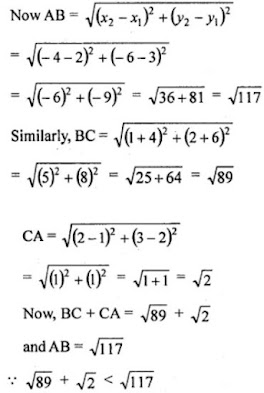∴ These points do not form a triangle.

13. The points A (2, 9), B (a, 5) and C (5, 5) are the vertices of a triangle ABC right angled at B. Find the values of a and hence the area of ∆ABC.

Solution

Given that, the points A (2, 9), B (a, 5) and C (5, 5) are the vertices of a ∆ABC right angled at B.
By Pythagoras theorem, AC² = AB² + BC² …(i)
Now by distance formula,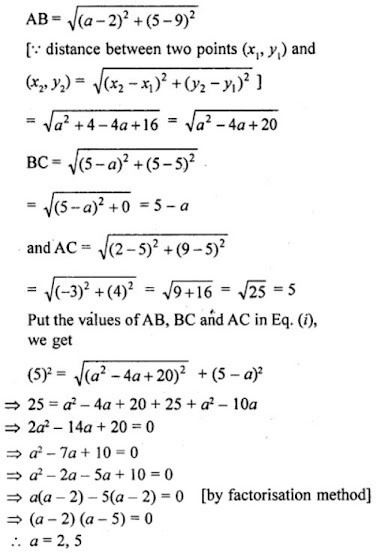Here, a ≠ 5, since at a = 5, the length of BC = 0. It is not possible because the sides AB, BC and CA from a right angled triangle.
Now, the coordinates of A, B and C becomes (2, 9), (2, 5) and (5, 5) respectively.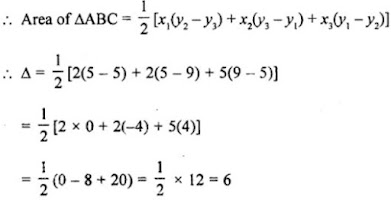Hence, the required area of ∆ABC is 6 sq. units.